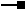# ITopologicalOperator2 Interface

## Members

Name DescriptionBoundary The boundary of this geometry. A polygon's boundary is a polyline. A polyline's boundary is a multipoint. A point or multipoint's boundary is an empty point or multipoint.Buffer Constructs a polygon that is the locus of points at a distance less than or equal to a specified distance from this geometry.Clip Constructs the intersection of this geometry and the specified envelope.ClipDense Constructs the intersection of this geometry and the specified envelope; densifies lines in output contributed by the clipping envelope.ClipToDomain Clips the geometry to the domain of the spatial reference. Useful for ensuring that buffers can be fit within the spatial domain of the feature class to which they are being added.ConstructBuffers Constructs a set of buffers at various distances. More efficient than calling Buffer repeatedly on the same geometry. This method is intended for internal use only.ConstructUnion Defines this geometry to be the union of the inputs. More efficient for unioning multiple geometries than calling Union repeatedly.ConvexHull Constructs the convex hull of this geometry.Cut Splits this geometry into a part left of the cutting polyline, and a part right of it.Difference Constructs the geometry containing points from this geometry but not the other geometry.Intersect Constructs the geometry that is the set-theoretic intersection of the input geometries. Use different resultDimension values to generate results of different dimensions.IntersectMultidimension Constructs the set-theoretic intersection of the inputs. The results are returned in a geometry bag with one element per result dimension.IsKnownSimple Indicates whether this geometry is known (or assumed) to be topologically correct.IsKnownSimple Indicates whether this geometry is known (or assumed) to be topologically correct.IsSimple Indicates whether this geometry is known (or assumed) to be topologically correct, after explicitly determining this if the geometry is not already known (or assumed) to be simple.QueryClipped Redefines clippedGeometry to be the intersection of this geometry and the clipping envelope.QueryClippedDense Redefines clippedGeometry to be the intersection of this geometry and the clipping envelope; densifies lines in the output contributed by the clipping envelope.Simplify Makes this geometry topologically correct.SymmetricDifference Constructs the geometry that contains points from either but not both input geometries.TopologyCache Provides a handle to the TopologyCache.Union Constructs the geometry that is the set-theoretic union of the input geometries.

### ITopologicalOperator2.ClipToDomain Method

Clips the geometry to the domain of the spatial reference. Useful for ensuring that buffers can be fit within the spatial domain of the feature class to which they are being added.

``````Public Sub ClipToDomain ( _
)
``````
``````public void ClipToDomain (
);
``````

### ITopologicalOperator2.ConstructBuffers Method

Constructs a set of buffers at various distances. More efficient than calling Buffer repeatedly on the same geometry. This method is intended for internal use only.

``````Public Function ConstructBuffers ( _
ByVal numBuffers As Integer, _
ByRef distances As Double _
) As IEnumGeometry
``````
``````public IEnumGeometry ConstructBuffers (
int numBuffers,
ref double distances
);
``````

#### Remarks

use the generic version of this method accesible through the GeometryEnvironment singleton object via the IGeometryBridge interface.

This method is only implemented for polygons and polylines.

``````When using C# you must use the IGeometryBridge interface to call this method.
``````
``````When using VBNET you must use the IGeometryBridge interface to call this method.
``````

### ITopologicalOperator2.IntersectMultidimension Method

Constructs the set-theoretic intersection of the inputs. The results are returned in a geometry bag with one element per result dimension.

``````Public Function IntersectMultidimension ( _
ByVal other As IGeometry _
) As IGeometry
``````
``````public IGeometry IntersectMultidimension (
IGeometry other
);
``````

#### Remarks

This method only works with the following combination of geometries: Polygon with Polyline (and vice versa), Polyline with Multipoint (and vice versa), Polygon with Multipoint (and vice versa).When using this method to intersect a Polygon with a Polyline (or vice versa) the geometry returned will be a GeometryBag.

When using this method to intersect a Multipoint with a Polygon (or vice versa) or Multipoint with a Polyline (or vice versa) the geometry returned will be a Multipoint.

If the desired combination of geometries is not available on this function, use Intersect on ITopologicalOperator.

### ITopologicalOperator2.IsKnownSimple Property

Indicates whether this geometry is known (or assumed) to be topologically correct.

``````Public WriteOnly Property IsKnownSimple_2
``````
``````public void IsKnownSimple_2 {set;}
``````

### ITopologicalOperator2.IsKnownSimple Property

Indicates whether this geometry is known (or assumed) to be topologically correct.

``````Public WriteOnly Property IsKnownSimple_2
``````
``````public void IsKnownSimple_2 {set;}
``````

## Inherited Interfaces

Interfaces Description
ITopologicalOperator Provides access to members for constructing new geometries based upon topological relationships between existing geometries.

## Classes that implement ITopologicalOperator2

Classes Description
MultiPatch A collection of surface patches.
Multipoint An ordered collection of points; optionally has measure, height and ID attributes.
Polygon A collection of rings ordered by their containment relationship; optionally has measure, height and ID attributes.
Polyline An ordered collection of paths; optionally has measure, height and ID attributes.

## Remarks

ITopologicalOperator2 methods must be applied on high-level geometries only. High-Level geometries are point, multipoint, polyline and polygon. To use this interface with low-level geometries such as segments (Line, Circular Arc, Elliptic Arc, Bezier Curve), paths or rings, they must be wrapped into high-level geometry types.

For multipatch geometries, generally the footprint or envelope is used.